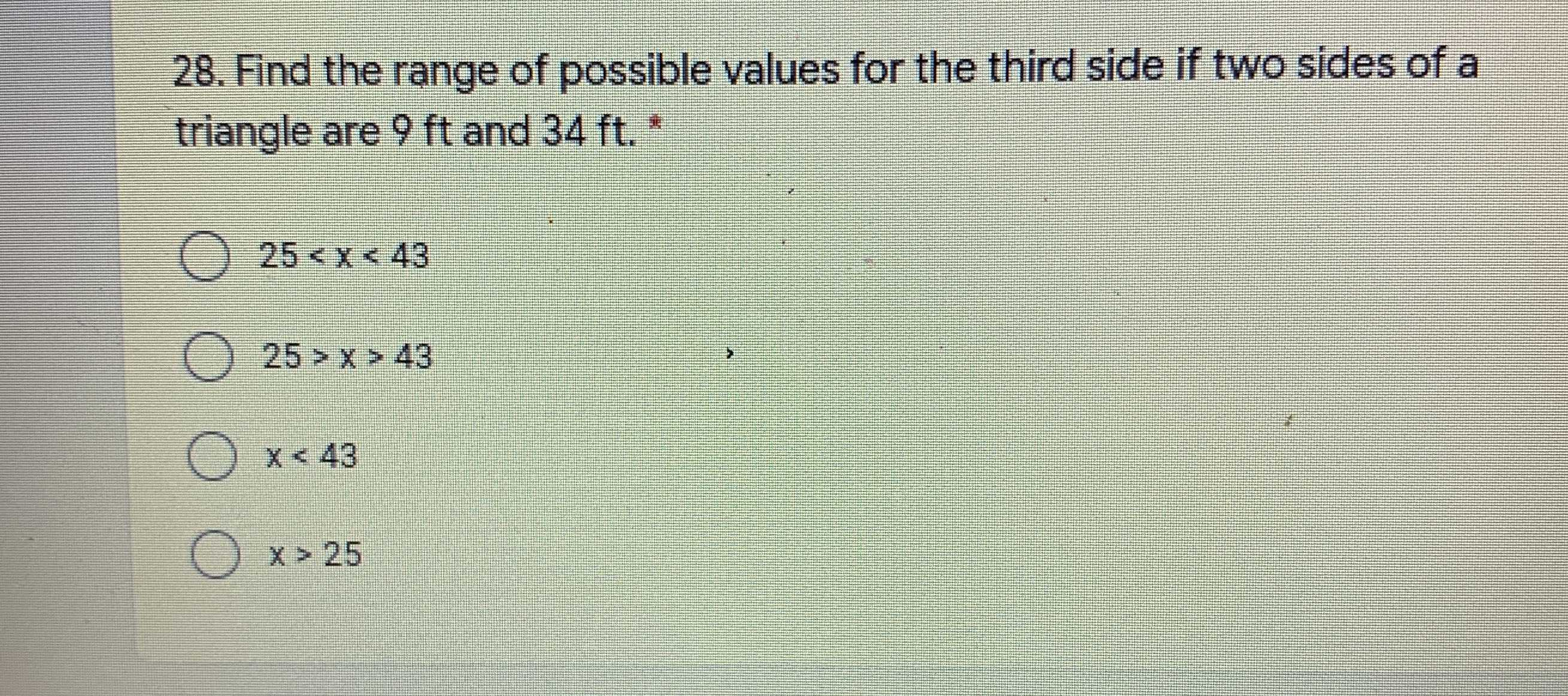### ¿Todavía tienes preguntas de matemáticas?

Pregunte a nuestros tutores expertos
Algebra
Pregunta28. Find the range of possible values for the third side if two sides of a triangle are $$9 ft$$ and $$34 ft$$ .

$$\ 25 < x < 43$$

$$25 > x > 43$$

$$x < 43$$

$$x > 25$$